# Understanding The Chain Rule

In this blog on “Understanding the chain rule,” we will learn the math behind the application of chain rule with the help of an example.

For those of you who are interested in Neural Networks and Deep Learning, the process of backpropagation is a very important concept which is extensively used while creating these advanced models. While performing backpropagation, we use the concept of chain rule to backpropagate the error values in prediction to adjust the weights.

To be able to understand this unit, you should know what a derivative is.

##What is a derivative?

Don’t sweat it, in case you don’t know or don’t remember the same, you can learn about it on the glossary section of Quantra website.

##What is the Chain Rule?

The chain rule is basically a formula for computing the derivative of a composition of two or more functions.

##Understanding the Chain Rule

Let us say that f and g are functions, then the chain rule expresses the derivative of their composition as f ∘ g (the function which maps x to f(g(x)) ). The derivative of this composition is calculated as mentioned below.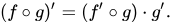Here f is the function of g and g is a function of variable x.

Another way of writing the above rule: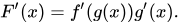Where the function F represents the composite function f(g(x))

Let us say that we have three variables x, y and z such that, the variable z depends on the variable y, which in turn depends on the variable x. So y and z are dependent variables, and z, via the intermediate variable of y, depends on x. Then the chain rule for differentiating the variable z may be written in the following manner.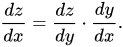This is the final formula that we use in backpropagation.

Here z is the function of y,

z = f(y)

and y is a function of x,

y= g(x)

Using the previous formula, we can rewrite the differential equation as follows: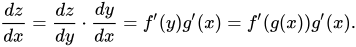Let us understand this better with the help of an example.

##Example of Chain Rule

Let us understand the chain rule with the help of a well-known example from Wikipedia. Assume that you are falling from the sky, the atmospheric pressure keeps changing during the fall. Check out the graph below to understand this change.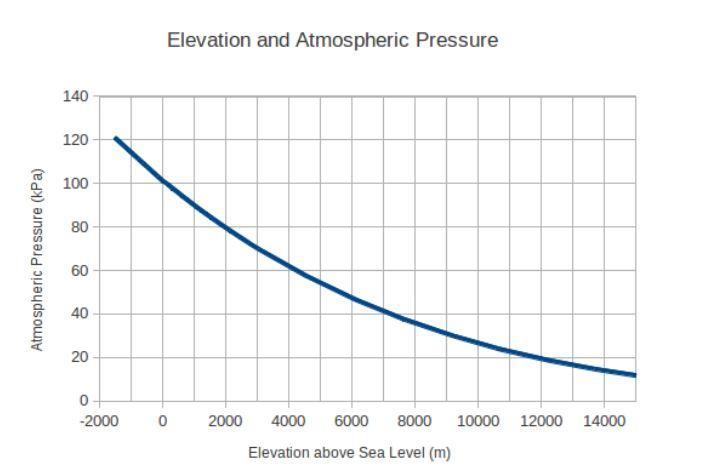At the time of your fall, 4000 meters above sea level, the initial velocity was zero, and the gravity is 9.8 meters per second squared. Now compare this situation to the previous chain rule equation. Let us say that the variable x in the equation is variable t, or time.

Then the variable y or g(t), which is the distance travelled by you since the beginning of the fall is given by

g(t) = 0.5*9.8t2

So, the height from the mean sea level can be given by the variable h, which is

h = 4000 - g(t)

Let us say that we also know, based on a model, the atmospheric pressure at a height h as:

f(h) = 101325 e−0.0001h

These two equations can be differentiated by their respective variable to get the following information:

g′(t) = −9.8t,

where, g′(t) is the velocity of you at time t

f′(h) = −10.1325e−0.0001h

where, f′(h) is the rate of change in atmospheric pressure with respect to height h

Now let us understand how we can combine these two equations to derive the

the rate of change in the atmospheric pressure with respect to time at t seconds after the skydiver's jump, using the chain rule: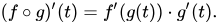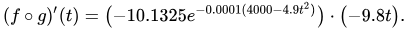This equation gives us the rate of change of atmospheric pressure with respect to time since fall. In neural networks, we will need to calculate the change in weights at each neuron with respect to the errors in prediction. As you might have imagined by now, the chain rule helps adjusts these weights accordingly.

## Conclusion

If we want to apply the chain rule to backpropagate the error in neural networks, then we will be using an equation such as this.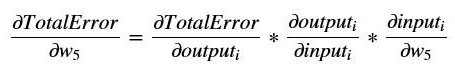In the Quantra’s course on Deep Learning in Trading with Dr. E. P. Chan, we will help you not only understand advanced concepts such as deep learning, but also apply them in the context of trading.

Disclaimer: All investments and trading in the stock market involve risk. Any decisions to place trades in the financial markets, including trading in stock or options or other financial instruments is a personal decision that should only be made after thorough research, including a personal risk and financial assessment and the engagement of professional assistance to the extent you believe necessary. The trading strategies or related information mentioned in this article is for informational purposes only.